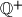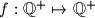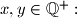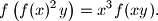« Vrati se

#### IMO Shortlist 2010 problem A5

Denote by$\mathbb{Q}^+$ the set of all positive rational numbers. Determine all functions$f : \mathbb{Q}^+ \mapsto \mathbb{Q}^+$ which satisfy the following equation for all$x, y \in \mathbb{Q}^+:$Proposed by Thomas Huber, Switzerland